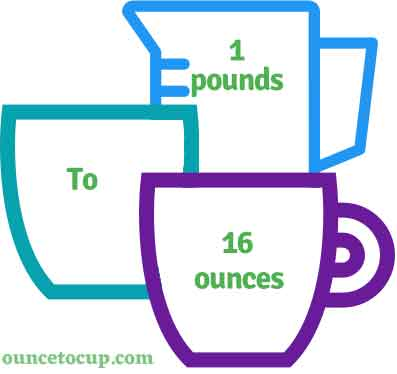# Pounds to Ounces (lbs to oz conversion)

Are you cooking your favorite dish? The detailed chart in the recipe includes the calculation of the 1 pound to ounce conversion.  Don't worry; use this calculator to determine how many 1 pound equals ounces in a minute.  This 1 lbs to oz converter gives an exact measurement for any recipe you prepare.

Pound Value:

lbs

Ounce Value:

oz

1 Pound = 16 Ounce
(1 lbs = 16 oz)

Try our auto 1 Pound to Ounce Calculator (Without Convert Button), Just change the first field value and you got final value.## How many oz is a lbs?

We know that the mass value of 1 lbs is equal to 16 oz. If you want to convert 1 lbs to an equal number of oz, just multiply the mass value by 16 or divide the value by 29.5735. Hence, 1 pound is equal to 16 oz.

The Answer is: 1 US Pounds = 16 US Ounce

1 lbs = 16 oz

Many of them try to search or find an answer for what is 1 pounds in oz? So, we’ll start with 1 lbs to oz conversion to know how big is 1 lbs.

## How To Calculate 1 lbs to oz?

To calculate 1 pounds to an equal number of ounces, simply follow the steps below.

Pounds to Ounces formula is:

Fluid Ounce = Pound * 16

Assume that we are finding out how many oz were found in 1 fl lbs of water, multiply by 16 to get the result.

Applying to Formula: oz = 1 lbs * 16 = 16 oz.

## How To Convert lbs to oz?

• To convert pounds to oz,
• Simply multiply the pound value by 16
• Applying to the formula, oz = 1 pounds * 16 [1x16].
• Hence, 1 pounds is equal to 16 oz.

## Some quick table references for pounds to ounce conversions:

Pounds [lbs]Ounce [oz]
1 lbs16 oz
2 lbs32 oz
3 lbs48 oz
4 lbs64 oz
5 lbs80 oz
6 lbs96 oz
7 lbs112 oz
8 lbs128 oz
9 lbs144 oz
10 lbs160 oz
11 lbs176 oz
12 lbs192 oz
13 lbs208 oz
14 lbs224 oz
15 lbs240 oz

## Reverse Calculation: How many pounds are in a oz?

• To convert 1 oz to lbs,
• Simply divide the 1 oz by 16.
• Then, applying the formula, lbs = 1 oz / 16 [1/16 = 0.0625].
• Hence, 1 ounce is equal to 0.0625 pound.